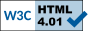Abstract Measures of
Low-Level Computational Complexity

The project Abstract Measures of Low-Level Computational Complexity (Principle Investigator: Dr Arnold Beckmann), which had been funded by the EPSRC grant EP/D03809X/1, has now come to an end.

# Overview

Assume you are an employee of a university who has to deal with assigning accommodation to new students. This year, for 400 new students only 100 free places are available in the dormitories. And to complicate matters, you have been given a list of pairs of students from your Dean with the requirement that no pair from this list appears in your final choice. This is an example of what a computer scientist calls an NP-problem, as it is easy to check whether a given choice of 100 students satisfies the requirement, but it is very hard to find them. The naive way of searching for a solution would be to test for all possibilities. But this way is totally hopeless as the number of possibilities is of unimaginably huge size - it is greater than the numbers of atoms in the known universe! Does this mean that we will never be able to compute the exact solution to the student assignment problem? Or, can it be that some clever computer scientist may find a different program which solves this problem in an efficient way?

The P vs NP problem asks exactly this question: NP is the class of all problems for which it is easy to check whether a given choice is a solution, and P those where in addition a solution can be effectively found. If we are able to show that the student assignment problem cannot be solved efficiently, then P is different from NP. If, however, a clever computer scientist finds an efficient program solving the student assignment problem, then P = NP. The P vs NP problem is one of the most challenging open questions in mathematics and computer science, which has been open now for more than thirty years. For example, the Clay Mathematics Institute has listed this problem in their collection of seven Millennium Problems whose solution is worth one millions US Dollars, see http://www.claymath.org/millennium/.

An approach to tackle the P vs NP problem is to employ mathematical logic. This approach uses deep connections between proving and computing which have been studied and are still studied since the early days of mathematical logic and computer science. The task of proving that for certain constellations of students a solution satisfying the Dean's requirements exists, and the task of actually computing such a solution, are intimately connected: The more mathematical resources are needed for proving the existence of a solution, the more computational resources are needed for computing a solution. Mathematical resources are what a mathematician usually calls axioms, and a collection of axioms is denoted axiom system. One well-studied collection of axiom systems related to low-level computational complexity like P and NP, is denoted Bounded Arithmetic.

In this project we have exploited properties of mathematical proofs to show that known abstract measures for proof strength of axioms of Bounded Arithmetic also provide characterisations of their computation strength. This has lead to very fruitful new approaches to study so-called definable search problems in Bounded Arithmetic which is currently a hot topic in the area of Bounded Arithmetic. Using our new methods we have identified new search complexity classes as variants of Polynomial Local Search Problems, which characterise exactly the definable search problems in Bounded Arithmetic. Based on these we have identified new generic problems which are conjectured to separate levels of Bounded Arithmetic and related propositional proof systems - a proof of this conjecture would solve a long standing open problem in Bounded Arithmetic. The investigation of this conjecture is ongoing research and will be the topic for future projects.

 websites: Arnold Beckmann 2014-03-14